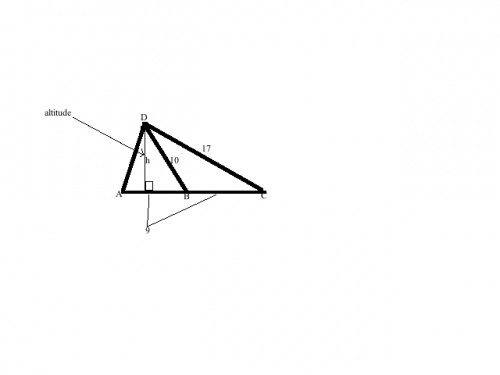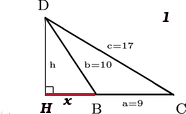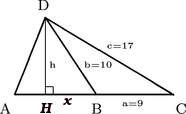# Homework help

## Recommended Posts

I have been trying to do this for a while and have no idea how, all I was given was that line DB is the median of line AC, I need to find the are of the triangles ADB and DCB, thanks for any help you canEdited by Niomus
##### Share on other sites

sorry double posted, other one is the real one, thanks

Edited by Niomus
##### Share on other sites

The image isn't showing in this thread, but going by the image in your other thread, it appears you were also given the lengths of several sides (namely of lines AB, BC, BD, and CD). Have you learned about Heron's formula and the properties of triangle medians? What have you tried so far?

Edited by John
##### Share on other sites

no we have not learned that yet, thank you, any Idea on how to find the area of triangle ADB? thanks

and we did learn the properties of a median of a triangle

##### Share on other sites

Try using the Law of Cosines to find the angles and the lengths of the sides that aren't given.

##### Share on other sites

Alright, well, think about the properties of triangle medians. What do they tell us about the relationship between triangles ABD and BCD in this case?

Also, since it's for a geometry class, I'm assuming we don't want to invoke trigonometry.

Edited by John
##### Share on other sites

the fact that it is a meridian tells me that line AB and BC are equal, other than that not much, this is the first geometry class I have taken and we are still pretty unknowledgeable of most things, we haven't learned cosines yet, thank you

##### Share on other sites

Hm, alright. Well, as it turns out, a median of a triangle divides the triangle's area in half. This means the areas of triangles ABD and BCD are equal. You know the lengths of all three sides of triangle BCD, which means you can plug them into Heron's formula (linked above) to determine the area of BCD.

I just don't know if your teacher will like this method, since you haven't learned about the formula yet.

##### Share on other sites

This can be done using very basic geometry - but it is very basic geometry that has been forgotten once we had the cosine rule.

If we go back to the founding tome of geometry - euclid's elements (and it does not get more basic than that) we find prop 12

Proposition 12

In obtuse-angled triangles the square on the side subtending the obtuse angle is greater than the squares on the sides containing the obtuse angle by twice the rectangle contained by one of the sides about the obtuse angle, namely that on which the perpendicular falls, and the straight line cut off outside by the perpendicular towards the obtuse angle

What this means in terms of our problem (let H be the point at which the perpendicular touches AB) is that

CD2 = BC2 + DB2 +2BC.BH

Where HB is the distance from B to where the perpendicular crosses AB

This is a formula with only one unknown - ie BH and thus you can solve for it. Hint it comes out as a nice whole number (always a pleasant relief)

Once you know BH you can use Pythagoras to work out the perpendicular height (HD) of the triangle HDC

You then have the base AC and the perpendicular height of of triangle ADC

Half base times perpendicular height is area.

Sorted. Although having to go back to Euclid is a bit odd.

If you cannot follow this - let me know and I will post my diagram

##### Share on other sites

yeah I could probably work it out but I would probably make a large amount of mistakes, if you could post the diagram it would be very helpful, thank you

##### Share on other sites

Thank you, imatfaal. I didn't know about that.You'll arrive at the same answer using either method, so use whichever one works best for you. Good luck.Edit: I'm assuming imatfaal will provide a setup for Euclid's method, but using Heron's formula, I'll set this up (also because I'm learning LaTeX and this'll be good practice):

Here is your triangle, drawn with the variables next to the sides I associated them with:

$\setlength{\unitlength}{1.5mm} \begin{picture}(30,30) \thicklines \put(2,3){\line(2,5){4}} \put(2,3){\line(10,0){20.75}} \put(6,13){\line(5,-3){16.75}} \put(6,13){\line(2,-3){6.6}} \thinlines \put(6,3){\line(0,1){10}} \put(6,4){\line(1,0){1}} \put(7,3){\line(0,1){1}} \put(0,0.5){\footnotesize A} \put(12,0.5){\footnotesize B} \put(23,0.5){\footnotesize C} \put(5,14){\footnotesize D} \put(14,9){\tiny c=17} \put(11,6.5){\tiny b=10} \put(6,1.5){\tiny 9} \put(16,1.5){\tiny a=9} \put(6.5,6.5){\tiny h} \end{picture}$

Heron's formula states that the area of a triangle is equal to

$\sqrt{s(s-a)(s-b)(s-c)}$

where

$s = \frac{a+b+c}{2}$

Hopefully that's not giving too much away.

Edited by John
##### Share on other sites

The Cosine Rule allows you to find the angle of from the three sides of a triangle but before this (ie before trigonometry sines/cosines etc) there were two rules one could use in these circumstances that allowed you to work around. These are Prop 12 and prop 13 of Euclids Elements - 12 applies to obtuse triangle and 13 to acute triangles

If we redraw the original triangle of which we know the side lengths whilst keeping the names we end up with the triangle BDC in blackProposition 12 tells us that if we extend one of the sides adjacent to B which is the large angle (ie x or HB in red) and drop a perpendicular from the other angle (ie h or DH in blue) that we can relate the length of the long side to the length of the two shorter sides (ie like pythagoras) but with a little addition

CD2 = BC2 + DB2 +2BC.BH

or following the diagrams names

c2=a2 + b2 +2ax

You will note this is very similar to the cosine rule c2 = a2 + b2 - 2abCosB (per the above names) note that Cos of an obtuse angle will be -ve and this will end up adding

c2=a2 + b2 +2ax This equation has only one unknown - ie x

Once you have solved for x you can go back to your full diagramYou can now look at the right-angled triangle made by DCH. We know side DC is c = 17. We know side CH is a + x. Via pythagorus we can now work out side HD or h .

Once we know h we can say as follows - have a triangle ADC the base is AC (which is given as 18) the perpendicular height is HD (which is h and we have just worked out) The area of the triangle ADC is one half base time height Area=1/2.18.h

WE know that medians halve the area of the triangle so we now know the area of the two triangles BDC and ADB which make up the large one .

##### Share on other sites

There is also an intuitive way.

Because there are no many known triangles with all 3 sides composed of integers, like the BCD triangle here with sides 9 17 10 respectively.

Have you learned the 3 4 5 triangle?

------------------

I guess your professor will subjugate the classroom prooving in 2 steps that h = 8.

Then that the surface of BCD is equal to its perimeter.

He must have read MacHale, D., "That 3,4,5 triangle again," Mathematical Gazette 73, March 1989, 14-16.

Edited by michel123456
##### Share on other sites

Michel - I have learnt that you think in different ways (but def not incorrect ways) reading your posts over the last year or so - but I still cannot see how to get h=8 in two steps.

Although through reading your post I do see how to solve this quandary in much the easiest way that I can find (or at least explain my solution through Euclid in terms of pythag) . I will not go to end of cal to avoid being too helpful - but please tell me if this is what you envisaged

1. 172=(x+9)2+h2 - Pythag large right triangle

2. 102 = x2+h2 - Pythag small right triangle

1. 289 = x2 + 18x + 81 +h2

1. 289 = 18x +81 + (x2 + h2)

1. 289 = 18x +81+ (100) ie sub in eq 2 for x2+h2 gives equation in terms of x. once you have x you can get h

This is exactly what I did above - but so much simpler as it does not require going back to arcane rules (other than pythag!)In fact it shows how once you have proved pythagoras then proposition 12 and 13 of euclid must follow - which is very neat.

Edited by imatfaal
##### Share on other sites

Michel - I have learnt that you think in different ways (but def not incorrect ways) reading your posts over the last year or so - but I still cannot see how to get h=8 in two steps.

Although through reading your post I do see how to solve this quandary in much the easiest way that I can find (or at least explain my solution through Euclid in terms of pythag) . I will not go to end of cal to avoid being too helpful - but please tell me if this is what you envisaged

1. 172=(x+9)2+h2 - Pythag large right triangle

2. 102 = x2+h2 - Pythag small right triangle

1. 289 = x2 + 18x + 81 +h2 sub in eq 2 for x2+h2 gives equation in terms of x. once you have x you can get h

This is exactly what I did above - but so much simpler as it does not require going back to arcane rules (other than pythag!)

No, I simply made a sketch at scale and the 3 4 5 triangle came out quite naturally.

I have learned to always try to make a sketch of any problem, even for non geometrical ones. In many case you see the solution very quickly. The algebraic way to get there has almost no interest to me: when you know the start and you know the end, the travel is only a matter of work and patience.

edit.

And also I cheated & got help from wiki after searching for integer triangle

edit2

I also tried to solve by adding one unit on the AB side & one unit on the BC side. Then you get a new triangle A' C' D inscribed in a circle of radius 10, the new angle at D is orthogonal by definition. The small added triangles have a surface of 1h. But I could not get further, the 345 triangle was much easier.

After 15 min. if I don't find a solution, I quit. No patience anymore.

##### Share on other sites

I was finally able to ask my teacher and learned that with what we know the answer is impossible to find, thank you all for all your help

##### Share on other sites

Well, that's anticlimactic.The methods described so far will provide you with the answer, but it's good that you got your teacher's input. Have fun with the rest of your geometry class, and feel free to ask any other questions that might come up as you progress.##### Share on other sites

Hey Hey Niomus - not so fast! Surely you know Pythagoras and how to solve a very equation! I think your teacher is wrongFrom the top, written out in absolute full, and with no spoilers

By Pythagoras

Triangle DCH is a Right-angled triangle therefore

1. c2=h2+(a+x)2 - rearrange and expand to give

1. c2=h2 +a2 + 2ax +x2 - inset known quantities ( ie a, b, c) to give

1. 289 = h2 + 81 + 18x + x2 - rearrange again to give

1. 208 -18x = h2 + x2

By Pythagoras

Triangle DBH is a Right-angled triangle therefore

2. b2 = h2 +x2 - inserting known quantities (ie b) gives

2. 100 = h2 + x2

You will note that the Righthand side of both equation 1 and equation 2 are the same (h2 + x2) and we can thus say that the two lefh hand sides must be equal and create eq #3

3. 208 -18x = 100 - rearrange to give

3. 108 = 18x - divide through

3. x = 6

Putting this answer for x into either equation #1 or #2 will give us the answer for h - let's use both to be sure

Eq #1

1. 289 = h2 + 81 + 18x + x2 - insert x=6

1. 289 = h2 + 81 + 18(6) + 62

1. 289 = h2 + 81 + 108 + 36

1. 289 - 108- 36 - 81 = h2

1. 64 = h2

1. h = 8

Eq #2

2. 100 = h2 + x2 - insert x=6

2. 100 = h2 + 62

2. 100 - 36 = h2

2. 64 = h2

2. h = 8

Ok both ways agree that h=8

The base of your triangle ADC is 18 units (that's a given) and the perpendicular height is h = 8 units

Area = 1/2 base X perp height

Area = 1/2 (18 X 8)

Area = 72 square units

We know that the median of a triangle divides into two smaller triangles of equal area - thus the area of ADH = DCH = 72/2 = 36 square units

All done using just pythagoras and simple algebra . BTW I would not raise this in class - cos teachers never like being called on something like this in class, but if you can understand it well enough to explain to your teacher (in private) then you will have learnt a nice little fact about how geometry can show quite complicated things with simple ideas.

Edited by imatfaal
##### Share on other sites

Well done.

BTW it looks to me as a method to find the height of any triangle of known sides without Heron's formula.

Edited by michel123456
##### Share on other sites

Shew, this's the first one that so struck me difficult to help on without being too suggestive, actually doing the work for the op, however you manage skirting it, *kudos.*

## Create an account

Register a new account